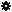## FCurveKey

Object Hierarchy | Related C++ Class: FCurveKey

SIObject

FCurveKey

v1.0

### Description

The FCurveKey represents a key on an FCurve and is used to access and modify information about that key.

### Methods

 GetConstraint2 IsClassOfIsEqualToSet

### Properties

 Application Categories Constraint FullNameHelp Index Interpolation Left LeftTanX LeftTanY Locked NameNestedObjects Origin OriginPath Parent Right RightTanX RightTanY Selected Time TypeValue

### Examples

#### 1. JScript Example

 ```/* This example demonstrates how to set an fcurve on the posx parameter and then how to access the keys through the FCurveKey object */ NewScene( null, false ) var oRoot = Application.ActiveProject.ActiveScene.Root; var oNull = oRoot.AddNull(); var oPosX = oNull.posx; // Create and connect an fcurve to the position x var oFCurve = oPosX.AddFCurve2( new Array(1, 10, 50, 0, 100, 10) ); // Set the zero slopes on the key tangents oFCurve.SetKeyTangents( new Array( -10.5, 0, 10.5, 0, -10.5, 0, 10.5, 0, -10.5, 0, 10.5, 0 )); // Get the keys on the fcurve for ( var i=0; i

#### 2. VBScript Example

 ```' ' This example demonstrates how to set an fcurve on the posx parameter ' and then how to access the keys through the FCurveKey object ' set oRoot = Application.ActiveProject.ActiveScene.Root set oNull = oRoot.AddNull set oPosX = oNull.posx ' Create and connect an fcurve to the position x set oFCurve = oPosX.AddFCurve2( Array( 1, 10, 50, 0, 100, 10 )) ' Set the zero slopes on the key tangents oFCurve.SetKeyTangents( Array( _ -10.5, 0, 10.5, 0, _ -10.5, 0, 10.5, 0, _ -10.5, 0, 10.5, 0 _ )) ' Get the keys on the fcurve for each oFCKey in oFCurve.Keys Application.LogMessage "oFCKey[" & oFCKey.Index & "] at frame " & oFCKey.Time & " = " & oFCKey.Value next ' Expected results: 'INFO : oFCKey at frame 1 = 10 'INFO : oFCKey at frame 50 = 0 'INFO : oFCKey at frame 100 = 10 ```

#### 3. Python Example

 ```# # This example demonstrates how to set an fcurve on the posx parameter # and then how to access the keys through the FCurveKey object # Application.NewScene( "", 0 ) oRoot = Application.ActiveProject.ActiveScene.Root oNull = oRoot.AddNull() oPosX = oNull.posx # Create and connect an fcurve to the position x oFCurve = oPosX.AddFCurve2( [1, 10, 50, 0, 100, 10] ) # Set the zero slopes on the key tangents oFCurve.SetKeyTangents( [ -10.5, 0, 10.5, 0, -10.5, 0, 10.5, 0, -10.5, 0, 10.5, 0 ]) # Get the keys on the fcurve for oFCKey in oFCurve.Keys : Application.LogMessage( "oFCKey[" + str(oFCKey.Index) + "] at frame " + str(oFCKey.Time) + " = " + str(oFCKey.Value) ) # Expected results: #INFO : oFCKey at frame 1.0 = 10.0 #INFO : oFCKey at frame 50.0 = 0.0 #INFO : oFCKey at frame 100.0 = 10.0 ```